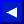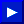### SGN

TYPE: Integer Function
FORMAT: SGN (<numeric>)

Action: SGN gives you an integer value depending upon the sign of the <numeric> argument. If the argument is positive the result is 1, if zero the result is also 0, if negative the result is -1.

EXAMPLE of SGN Function:
```   90 ON SGN(DV)+2 GOTO 100, 200, 300
(jump to 100 if DV=negative, 200 if DV=0, 300 if DV=positive)
```

### SIN

TYPE: Floating-Point Function
FORMAT: SIN (<numeric>)

Action: SIN gives you the sine of the <numeric> argument, in radians. The value of COS(X) is equal to SIN(x+3.14159265/2).

EXAMPLE of SIN Function:
```   235 AA=SIN(1.5):PRINT AA
.997494987
```

### SPC

TYPE: String Function
FORMAT: SPC (<numeric>)

Action: The SPC function is used to control the formatting of data, as either an output to the screen or into a logical file. The number of SPaCes given by the <numeric> argument are printed, starting at the first available position. For screen or tape files the value of the argument is in the range of 0 to 255 and for disk files up to 254. For printer files, an automatic carriage-return and line-feed will be performed by the printer if a SPaCe is printed in the last character position of a line. No SPaCes are printed on the following line.This page has been created by Sami Rautiainen. Read the small print. Last updated November 08, 1998.